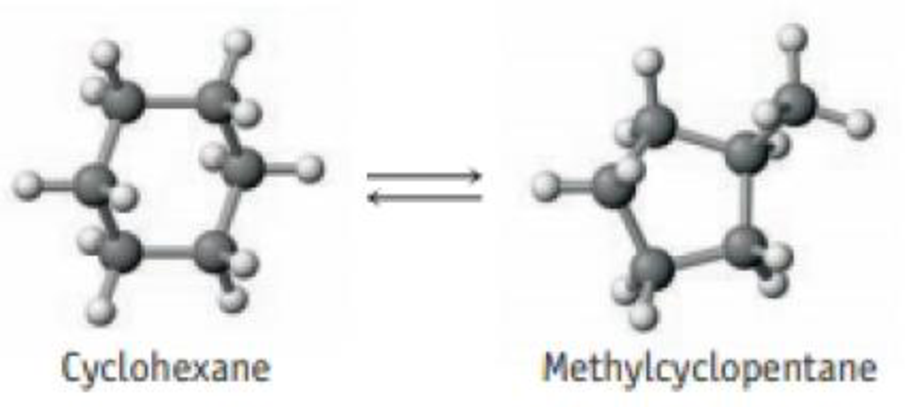Chapter 15, Problem 14PS

Chapter
Section
Textbook Problem

Cyclohexane, C6H12, a hydrocarbon, can isomerize or change into methylcyclopentane, a compound of the same formula (C5H9CH3) but with a different molecular structure.sssssThe equilibrium constant has been estimated to be 0.12 at 25 °C. If you had originally placed 0.045 mol of cyclohexane in a 2.8-L flask, what would be the concentrations of cyclohexane and methylcyclopentane when equilibrium is established?

Interpretation Introduction

Interpretation: The equilibrium concentration of cyclohexane C6H12 and methylcyclopentane C5H9CH3 when the system comes to equilibrium has to be determined.

Concept introduction:

• Equilibrium constant: At equilibrium the ratio of products to reactants has a constant value. And it is represented by the letter K.

For a general reaction, aA+bBcC+dD

The equilibrium constant Kc=[C]c[D]d[A]a[B]b, where a, b, c and d are the stoichiometric coefficients of reactant and product in the reaction. Concentration value for solid substance is 1.

If the value of Kc and the concentration of any of the reactant of a reaction is known then the concentration of product can be determined by multiplying Kc with the concentration of reactant.

• Concentration =AmountofsubstanceVolume
• ICE (reaction initial concentration equilibrium) table is mainly used to calculate the value of K for a reaction. This table contains the concentration of reactant and product in various stage of reaction.
Explanation

The given equilibrium set up contains cyclohexane C6H12 and methylcyclopentane C5H9CH3. And the value of Kc for the isomerization between these two compounds is 0.12at250C

The concentration of cyclohexane C6H12= 0.045mol2.8L=0.0160mol/L

By using these concentration ICE table for this reaction can be constructed as follows,

 Reaction                      C6H12              ⇄          C5H9CH3 Initial concentration (mol/L) 0

Still sussing out bartleby?

Check out a sample textbook solution.

See a sample solution

The Solution to Your Study Problems

Bartleby provides explanations to thousands of textbook problems written by our experts, many with advanced degrees!

Get Started